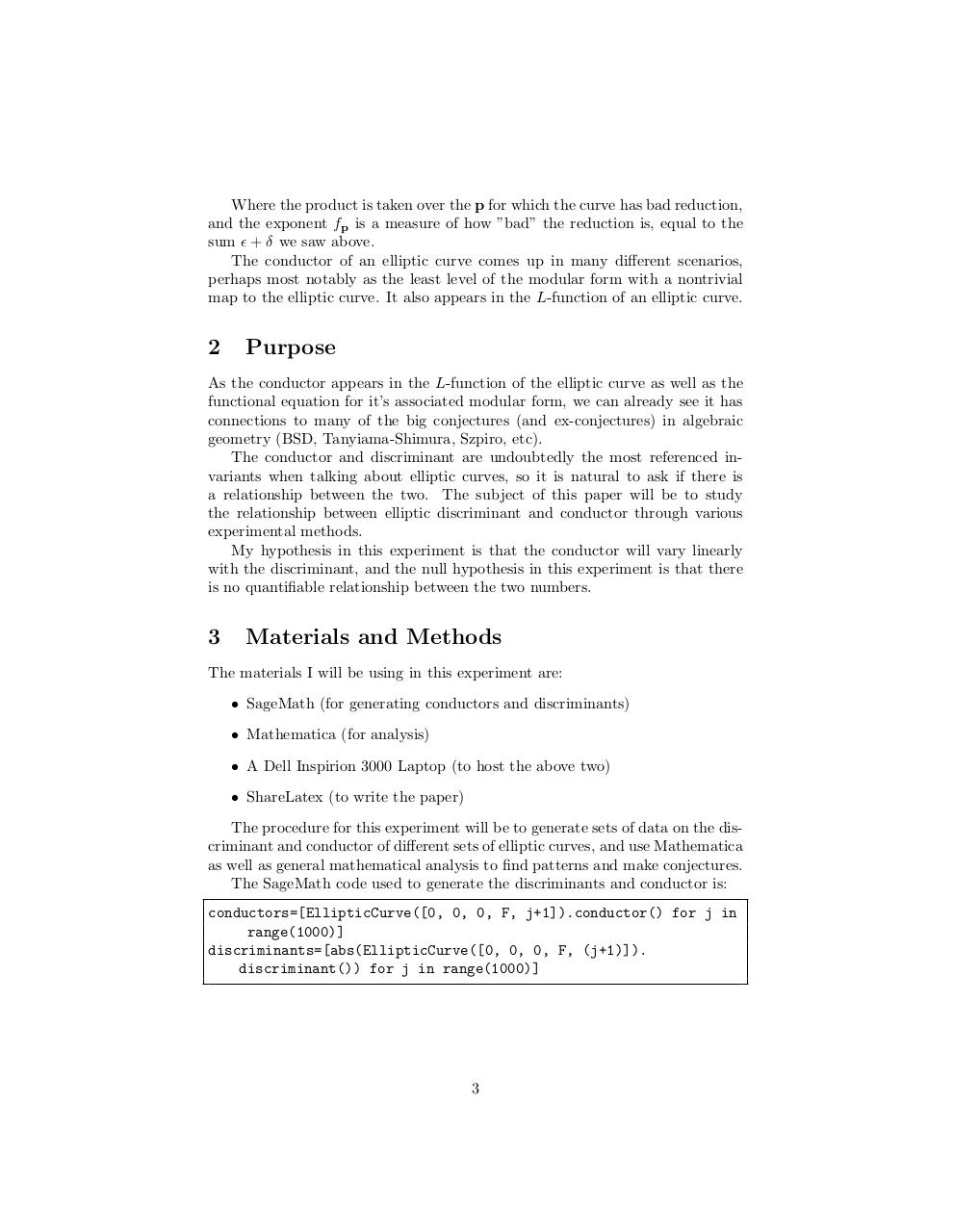# Sci Research (2).pdfPage 1 2 3 4 5 6 7 8 9

#### Text preview

Where the product is taken over the p for which the curve has bad reduction,
and the exponent fp is a measure of how ”bad” the reduction is, equal to the
sum  + δ we saw above.
The conductor of an elliptic curve comes up in many different scenarios,
perhaps most notably as the least level of the modular form with a nontrivial
map to the elliptic curve. It also appears in the L-function of an elliptic curve.

2

Purpose

As the conductor appears in the L-function of the elliptic curve as well as the
functional equation for it’s associated modular form, we can already see it has
connections to many of the big conjectures (and ex-conjectures) in algebraic
geometry (BSD, Tanyiama-Shimura, Szpiro, etc).
The conductor and discriminant are undoubtedly the most referenced invariants when talking about elliptic curves, so it is natural to ask if there is
a relationship between the two. The subject of this paper will be to study
the relationship between elliptic discriminant and conductor through various
experimental methods.
My hypothesis in this experiment is that the conductor will vary linearly
with the discriminant, and the null hypothesis in this experiment is that there
is no quantifiable relationship between the two numbers.

3

Materials and Methods

The materials I will be using in this experiment are:
• SageMath (for generating conductors and discriminants)
• Mathematica (for analysis)
• A Dell Inspirion 3000 Laptop (to host the above two)
• ShareLatex (to write the paper)
The procedure for this experiment will be to generate sets of data on the discriminant and conductor of different sets of elliptic curves, and use Mathematica
as well as general mathematical analysis to find patterns and make conjectures.
The SageMath code used to generate the discriminants and conductor is:
conductors=[EllipticCurve([0, 0, 0, F, j+1]).conductor() for j in
range(1000)]
discriminants=[abs(EllipticCurve([0, 0, 0, F, (j+1)]).
discriminant()) for j in range(1000)]

3